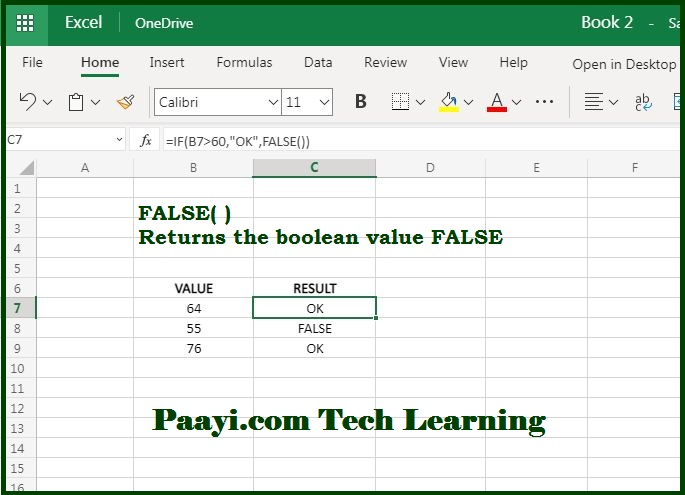# Learn How to Use Microsoft Excel FALSE Function

Written by | 0 Comments | 712 Views

In this article, you will learn how to use the Microsoft Excel FALSE function and its prime function in Microsoft Excel. You will also get to know the Microsoft Excel FALSE function return value and syntax with the help of some examples.

## Microsoft Excel FALSE Function

The Microsoft Excel FALSE function returns the value - "FALSE." It is classified as the compatibility function and is usually not needed in many cases. It is similar to using the Boolean Value FALSE directly in a formula. The purpose of this function is to generate value FALSE.

### Return Value of FALSE Function

The logical value FALSE

=FALSE( )

## How to Use Microsoft Excel FALSE Function?The FALSE function does not take any arguments and generates the Boolean value FALSE.  Let us understand this by the example given below:

To return value FALSE, if the value in A1 < 0, you can use this formula:

= IF (A1 < 0, FALSE( ) )

It should be noted that you can just enter the word FALSE directly into a cell or formula, and Microsoft Excel interprets it as a logical value FALSE. You can understand this by the examples given below:

Both these formulas are functionally identical:

= IF (A1 < 0, FALSE( ) )

= IF (A1 < 0, FALSE)

Both of these formulas will return the value FALSE, in case the value in A1 < 0.

According to Microsoft Excel, the FALSE function is labeled as a compatibility function in Microsoft Excel for compatibility with other spreadsheet applications.